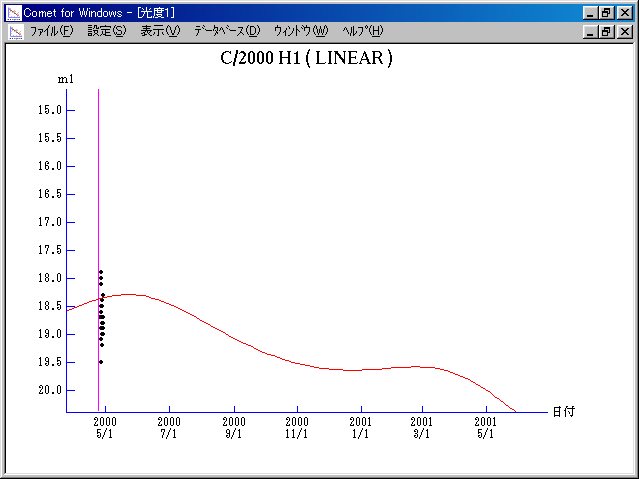# \$B%j%K%"WB@1(B

C/2000 H1 ( LINEAR )###\$B%W%m%U%#!<%k(B

 \$BH/8+F|(B 2000\$BG/(B4\$B7n(B26\$BF|(B \$BH/8+8wEY(B 18.7\$BEy(B \$BH/8+ Lincoln Laboratory Near-Earth Asteroid Research project

###\$B50F;MWAG(B

```   Improved parabolic orbital elements by K. Muraoka,
from 81 observations  2000 Apr. 26 to May 13,  m.e. = +/- 0".75.

T  =  2000 Jan. 28.4583  TT
Peri. =   78.7098
Node  =  356.4377   (2000.0)
Incl. =  118.2401
q  =    3.635720  AU
e  =    1.0
```

###\$B@1?^(B###\$B8wEYJQ2=(B

```        m1 = 9.5 + 5 log\$B&\$(B + 10.0 log r
```##### \$B50F;MWAG\$OB<2,7r<#;a\$N7W;;\$K\$h\$k\$b\$N\$G\$9!#(B \$B@1?^\$O(B StellaNavigator Ver.2.0 for Windows (\$B%"%9%H%m%"!<%D(B \$BJTCx(B / \$B%"%9%-!<=PHG6I4)(B) \$B\$G:n@.\$7\$?\$b\$N\$G\$9!#(B \$B8wEY%0%i%U\$O(BComet for Windows\$B\$G:n@.\$7\$?\$b\$N\$G\$9!#(B